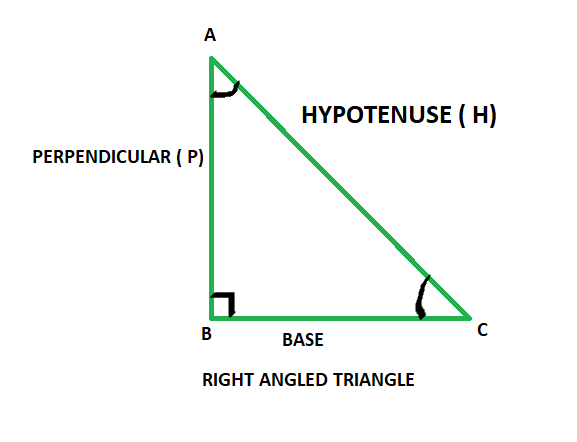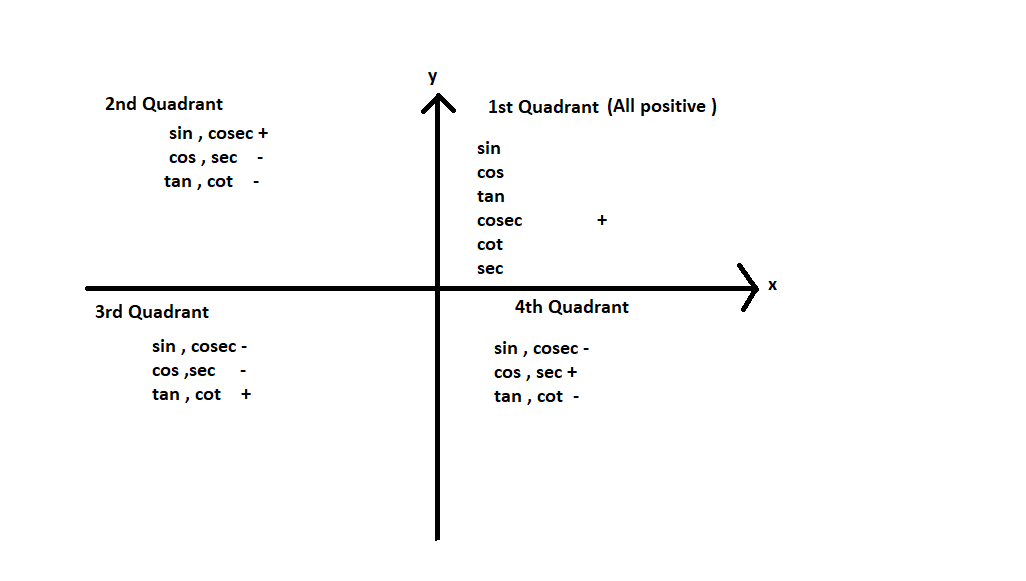GeeksforGeeks App
Open AppBrowser
Continue

# If sin (A – B) = 1/2, cos (A + B) = 1/2, and 0° B, then find the value of A and B

Trigonometry is a discipline of mathematics that studies the relationships between the lengths of the sides and angles of a right-angled triangle. Trigonometric functions, also known as goniometric functions, angle functions, or circular functions, are functions that establish the relationship between an angle to the ratio of two of the sides of a right-angled triangle. The six main trigonometric functions are sine, cosine, tangent, cotangent, secant, or cosecant.

Angles defined by the ratios of trigonometric functions are known as trigonometry angles. Trigonometric angles represent trigonometric functions. The value of the angle can be anywhere between 0-360°.As given in the above figure in a right-angled triangle:

• Hypotenuse: The side opposite to the right angle is the hypotenuse, It is the longest side in a right-angled triangle and opposite to the 90° angle.
• Base: The side on which angle C lies is known as the base.
• Perpendicular: It is the side opposite to angle C in consideration.

Trigonometric Functions

Trigonometry has 6 basic trigonometric functions, they are sine, cosine, tangent, cosecant, secant, and cotangent. Now let’s look into the trigonometric functions. The six trigonometric functions are as follows,

sine: It is defined as the ratio of perpendicular and hypotenuse and It is represented as sin θ

cosine: It is defined as the ratio of base and hypotenuse and it is represented as cos θ

tangent: It is defined as the ratio of sine and cosine of an angle. Thus the definition of tangent comes out to be the ratio of perpendicular and base and is represented as tan θ

cosecant: It is the reciprocal of sin θ and is represented as cosec θ.

secant: It is the reciprocal of cos θ and is represented as sec θ.

cotangent: It is the reciprocal of tan θ and is represented as cot θ.

According to the above image, Trigonometric Ratios are

Sin θ = Perpendicular / Hypotenuse = AB/AC

Cosine θ = Base / Hypotenuse = BC / AC

Tangent θ = Perpendicular / Base = AB / BC

Cosecant θ = Hypotenuse / Perpendicular = AC/AB

Secant θ = Hypotenuse / Base = AC/BC

Cotangent θ = Base / Perpendicular = BC/AB

Reciprocal Identities

Sin θ = 1/ Cosec θ                    OR        Cosec θ = 1/ Sin θ

Cos θ = 1/ Sec θ                       OR        Sec θ = 1 / Cos θ

Cot θ = 1 / Tan θ                     OR         Tan θ = 1 / Cot θ

Cot θ = Cos θ / Sin θ               OR         Tan θ = Sin θ / Cos θ

Tan θ.Cot θ = 1

Values of Trigonometric Ratios

Trigonometric Identities of Complementary and Supplementary Angles

• Complementary Angles: Pair of angles whose sum is equal to 90°
• Supplementary Angles: Pair of angles whose sum is equal to 180°

Identities of Complementary angles are

sin (90° – θ) = cos θ

cos (90° – θ) = sin θ

tan (90° – θ) = cot θ

cot (90° – θ) = tan θ

sec (90° – θ) = cosec θ

cosec (90° – θ) = sec θ

Identities of supplementary angles

sin (180° – θ) = sin θ

cos (180° – θ) = – cos θ

tan (180° – θ) = – tan θ

cot (180° – θ) = – cot θ

sec (180° – θ) = – sec θ

cosec (180° – θ) = – cosec θ### If sin (A – B) = 1/2, cos (A + B) = 1/2, and 0° < A + B ≤ 90°, A > B, then find the value of A and B

Solution:

We have

sin(A – B) = 1/2

Sin(A-B) = sin (30o)                                   [sin (30o) = 1/2]

then equate both the sides,

so we get A – B = 30o …(1)

And cso (A + B) = 1/2

⇒ cos(A + B) = cos (60o)                                 [cos (60o) = 1/2]

By equating both the sides,

we have  A + B = 60o …(2)

A – B = 30

+         A + B = 60o

we get 2A = 90o

⇒ A = 45o

Now, here Putting the value of A in Eq.(2),

45o + B =60o

⇒              B = 15o

Hence, the value of  A = 45o and B = 15o

### Similar Questions

Question 1: Prove that (cos θ sec θ)/cot θ = tan θ

Solution:

Here we have cos theta sec theta / cot theta = tan theta

Therefore { cos θ sec θ }/ cot θ = tan θ

By taking L.H.S

cos θ sec θ / cot θ

we can write cos θ sec θ as 1

= (cos θ sec θ )/cot θ

= 1/cot θ                            { Cos θ = 1/ Sec θ   therefore Cos θ Sec θ  = 1}

= tan θ                              { Tan θ = 1 / Cot θ }

Therefore LHS = RHS

{cos θ sec θ}/ cot θ = tan θ

Hence Proved

Question 2: Prove (1 + cot2θ) (1 – cos θ)(1 + cos θ) = 1

Solution:

We have LHS

= (1 + cot2θ) (1 – cos θ)(1 + cos θ)

= (1 + cot2θ) (1 – cos2 θ)

= cosec2θ sin2θ                                   {1 + cot2θ = cosec2θ  and 1 – cos2 θ  = sin2θ}

= (1/sin2θ) × sin2θ                              {Cosec2θ = 1/ Sin2θ}

= 1

= RHS

Hence Proved

My Personal Notes arrow_drop_up
Related Tutorials